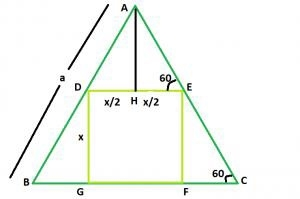# Biggest Square that can be inscribed within an Equilateral triangle in C?

An inscribed planar shape or solid is one that is enclosed by and "fits snugly" inside another geometric shape or solid. To say that "square is inscribed in triangle" means precisely the same thing as "triangle is circumscribed about square".

Biggest Square that can be inscribed within an Equilateral triangle −Biggest Square that can be inscribed within an Equilateral triangle −

Let’s take an example,

Input: 5
Output: 2.32

## Explanation

The side of the square be x.

Now, AH is perpendicular to DE.

DE is parallel to BC, angle AED = angle ACB = 60

In triangle EFC,

⇒ Sin60 = x/ EC

⇒ √3 / 2 = x/EC

⇒ EC = 2x/√3

In triangle AHE,

⇒ Cos 60 = x/2AE

⇒ 1/2 = x/2AE

⇒ AE = x

side AC of the triangle = 2x/√3 + x. Now,

a = 2x/√3 + x

x = a/(1 + 2/√3) = 0.464a

## Example

Live Demo

#include <stdio.h>
#include <math.h>
int main() {
float a = 5;
float area = 0.464 * a;
printf("The area is : %f",area);
return 0;
}

## Output

The area is : 2.320000